International Journal of Intelligent Information Systems
Volume 5, Issue 5, October 2016, Pages: 75-81

An Evolutionary Method of Neural Network in System Identification

Shuming T. Wang1, Chi-Yen Shen1, Yu-Ju Chen2, Chuo-Yean Chang3, Rey-Chue Hwang1, *

1Department of Electrical Engineering, I-Shou University, Kaohsiung City, Taiwan, R.O.C.

2Department of Information Management, Cheng Shiu University, Kaohsiung City, Taiwan, R.O.C.

3Department of Electrical Engineering, Cheng Shiu University, Kaohsiung City, Taiwan, R.O.C.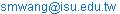(Shuming T. Wang)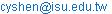(Chi-Yen Shen )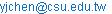(Yu-Ju Chen)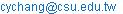(Chuo-Yean Chang)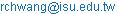(Rey-Chue Hwang)

*Corresponding author

Shuming T. Wang, Chi-Yen Shen, Yu-Ju Chen, Chuo-Yean Chang, Rey-Chue Hwang. An Evolutionary Method of Neural Network in System Identification. International Journal of Intelligent Information Systems. Vol. 5, No. 5, 2016, pp. 75-81. doi: 10.11648/j.ijiis.20160505.14

Received: September 19, 2016; Accepted: October 12, 2016; Published: October 20, 2016

Abstract: This paper presents an evolutionary method for calculating the important degree (ID) of individual input variable of well-trained neural network (NN). The importance of each input variable of neural network could be distinguished in accordance with ID value obtained. In this research, several linear and nonlinear systems’ identifications were firstly studied and simulated. From the simulation results shown, the evolutionary method proposed is quite promising and accurate for the estimation of system’s parameters. In other worlds, the method proposed could be used for data mining in the real applications. In order to verify our inference view, the evaporation process of thin film was studied either. It is a real case of industrial application. Again, the studied results show that the method proposed indeed has the superiority and potential in the area of data mining.

Keywords: Evolutionary, Important Degree, Neural Network, System Identification

Contents

1. Introduction

It is well known that system identification is a method which can identify the mathematical model of an unknown system from the measurements of system’s inputs and outputs. It has been employed into many areas such as industrial process, control system, economic forecasting, social science and so on. Many classes of system identification have been studied such as Volterra and Wiener series , NARMAX models  and neural network (NN) [3-7]. Generally, the steps like model hypothesis, estimation of parameters and system verification are indispensable for identifying an unknown system.

Recently, data mining has been widely used in the applications of signal processing and system identification. It is the process of analyzing data from different perspectives and then extracts the hidden information from a large data base. It can help the researcher to grasp the useful information that might be ignored and missed in the process of data analysis. The tasks of data mining generally include four classes which are classification, clustering, regression, and association rule learning [8-14]. In which, regression analysis consists of the graphic and analytic methods. It aims to obtain the relationships between response variable (output) and other predictor variables (inputs) for an unknown system. Its goal is to express the response variable as a function of the predictor variables. The major uses of regression methodology include model specification and parameter estimation . Model specification is a very important step in the application of system identification. Its objective is the assessment of the relative values of individual predictor variables on the prediction of the response. Basically, all relevant variables in the database must be analyzed in order to find which variable has the higher correlation with the response. Thus, the parameter estimation of the model is necessary to the regression analysis.

Due to the powerful learning and adaptive capabilities, NN model has been widely studied in the area of data mining. Many research articles have been proposed [16-22]. NN has the ability to deal with lots of linear or nonlinear modeling problems. Through the learning from data provided, NN is able to generate a mapping between input and output pairs bypassing the complicated statistical analysis steps. In general, the applications of NN model in data mining can be classified into four major categories: (1) Feed-forward NN, which is mainly used in the areas of prediction and pattern recognition; (2) Feedback NN, which is mainly used for associative memory and optimization calculation; (3) Self-organization NN, which is mainly used for cluster analysis; (4) Random NN, which has the advantages of associative memory and image processing .

In this research, the studies of NN versus regression analysis were studied. The assessment of the relative values of individual predictor variables (inputs) on the prediction of the response (output) was analyzed based on NN technique. The supervised learning feed-forward NN was mainly studied. And, the error back-propagation (BP) learning algorithm was used as the training rule of NN model. The details of NN structure and its learning algorithm are described in Section 2. Section 3 presents some relevant experiments and results. At last, a conclusion is given in Section 4.

2. NN Model and Its Learning Algorithm

The NN structure commonly known as multi-layered feed-forward net is used in this study. A three-layered feed-forward NN model as shown in Figure 1 is the selected topology. Each layer is connected to a layer above it in a feed-forward manner in the sense that no feed-back from the same layer or a layer above. All connections have a multiplying weight associated with them. Training is equivalent to find the proper weights for all connections such that a desired output is generated for a given input set.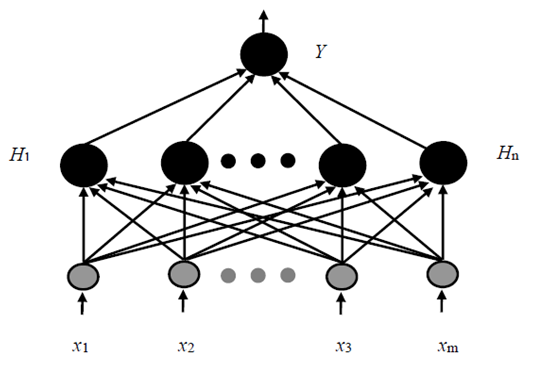Figure 1. A three-layered feed-forward neural network..

In NN model, the nonlinear activation function of all nodes is the sigmoid function. Its math form is expressed as the following equation and its diagram is shown in Figure 2.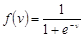(1)

Where,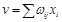,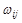is the strength of connection between node j and node i in the layer below and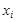is the value of node i.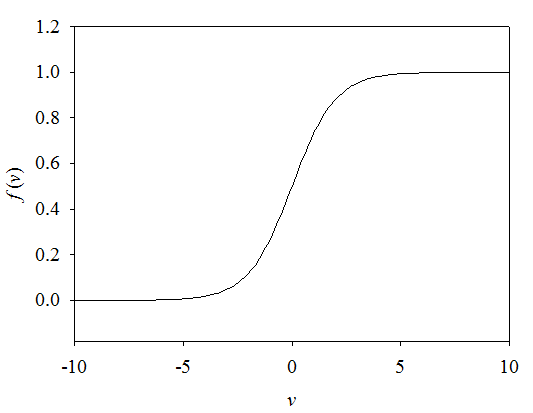Figure 2. The diagram of sigmoid function.

Here, we use a simple three-layered NN model to describe the evolutional method we proposed for calculating the important degree (ID) of individual input variable of a well-trained NN [24-25]. In Figure 1, it can be clearly found that the hidden nodes’ outputs (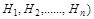and network output (Y) can be expressed as the following nonlinear math forms to the inputs (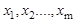).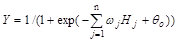(2)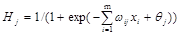(3)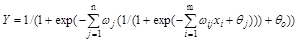(4)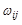is the strength of connection between hidden node j and input node i and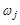is the strength of connection between hidden node j and output node Y.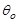and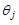are bias terms.

The sigmoid function is an increasing function which has the output values within the range [0,1], thus, the following relationships could be inferred.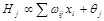(5)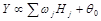(6)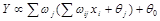(7)

According to the inferences of Eqs. (5), (6) and (7), the important degree (ID) and the percentage important degree (PID) of input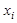to output Y are defined by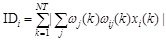(8)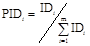(9)

where, NT is the total number of inputand m is the number (category) of input variables.

3. Experiments and Results

3.1. Linear and Nonlinear Regression Models

The aim of regression is to express the response variable as a function of the predictor variables. For linear regression, the response variable is supposed to have the linear relationship with the predictor variables. Denoting the response variable by Y and the m predictor variables by x1, x2, ……, xm, the linear relationship takes the form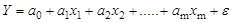(10)

In expression,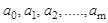are unknown parameters needed to be determined.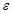is the noise term.

Nonlinear regression is a form of regression analysis in which the response variable is modeled by a function which is a nonlinear combination of the predictor variables. For instance, a nonlinear function is given as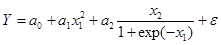(11)

In expression, the relationship between Y and (x1, x2) are nonlinear. In general, it is very difficult to obtain an exact closed-form expression for the unknown model in the nonlinear regression analysis. The data are usually fitted by a method of successive approximations. The present adaptive methods are very successful in linear regression. The application of these methods to nonlinear regression, however, requires several simplifications. And, only the approximate model could be obtained.

3.2. NN vs. Linear Regression Model

A linear regression model is given as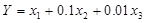(12)

The ratios of the coefficients among input variables are x1:x2=10:1, x1:x3= 100:1, and x2:x3=10:1. That means the degrees of importance of x1 to Y is 10 times x2 to Y, and 100 times x3 to Y, if x1, x2 and x3 have the same statistic distributions.

In our studies, Eq. (12) was firstly considered and 500 points were generated from the equation. Then, we assume this linear model is an unknown system which needs to be identified. In system’s identification process, five variables, x1, x2, x3, x4 and x5 are collected and assumed to be the possible influencing factors. Five variables are generated by uniformly distributed random numbers with mean zero and variance three and they have the values in the range of [3,3]. Table 1 lists the statistics of (x1, x2, x3, x4, x5).

Table 1. The statistics of (x1, x2, x3, x4, x5).

 Variable Range Mean Variance x1 -3~+3 -0.03718 3.01432 x2 -3~+3 0.06362 3.04612 x3 -3~+3 -0.01232 2.99183 x4 -3~+3 -0.09754 2.99003 x5 -3~+3 0.06445 3.02189

A NN with size m-4-1 was used to model this linear equation. The number of input nodes (m) of network is based on the variables used. In this study, 400 points were used for NN’s training and 100 points were used for testing. Table 2 lists the testing mean absolute errors (MAE) of NN modeling with different input variables. From the values of MAE, we conclude that NN has the efficient learning to this unknown system.

Table 2. The MAEs of NN modeling with different input variables.

 NN Size 3-4-1 4-4-1 5-4-1 Input Variables (x1,x2,x3) (x1,x2,x3,x4) (x1,x2,x3,x4,x5) MAE 0.004701 0.004845 0.004739

The corresponding IDi value of each input variable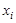in the well-trained NN models is then calculated. Table 3 is ID and PID values calculated for NN models with three, four and five inputs, respectively. From the table results shown, a phenomenon could be observed. For size 3-4-1 NN, the PID ratio of (x1, x2, x3) is (1:0.110:0.010). For size 4-4-1 NN, the PID ratio of (x1, x2, x3, x4) is (1:0.113:0.011:0.0017). For size 5-4-1 NN, the PID ratio of (x1, x2, x3, x4, x5) is (1:0.111:0.0107:0.00024:0.00135). It is clearly found that the PID ratios of (x1, x2, x3) in three NN models are all very close to the ratio (1:0.1:0.01) of the coefficients of (x1, x2, x3) in the original linear equation (12). The ID and PID values of x4 and x5 are much smaller than other variables’. This phenomenon shows that the nonlinear NN model is capable of evaluating the importance degree of each input variable to the output. And, x4 and x5 could be treated as the useless terms or noises due to their low ID and PID values.

Table 3. ID and PID values calculated by three NN models.

 NN Size 3-4-1 4-4-1 5-4-1 x1 ID 72.45134 72.48423 73.29393 (PID) (89.265%) (88.766%) (89.036%) x2 ID 7.99117 8.22012 8.12652 (PID) (9.846%) (10.067%) (9.872%) x3 ID 0.72148 0.82732 0.78352 (PID) (0.889%) (1.013%) (0.952%) x4 ID 0.12453 0.01704 (PID) (0.153%) (0.021%) x5 ID 0.09872 (PID) (0.12%)

In order to evidence the validity of the method we proposed, 500 points were regenerated. x1, x2, x3, x4 and x5 are uniformly distributed random numbers with different ranges. Table 4 lists the statistics of (x1, x2, x3, x4, x5).

Table 4. The statistics of (x1, x2, x3, x4, x5).

 Variable Range Mean x1 -1~+1 -0.00247 x2 -10~+10 -0.14673 x3 -5~+5 0.16588 x4 -1~+1 -0.01398 x5 -5~+5 -0.03153

From the statistics of Table 4, we conclude that the average absolute value of x2 should be 10 times the average absolute value of x1 and the average absolute value of x3 should be 5 times the average absolute value of x1. Multiplying the coefficient of each variable in Eq. (12), the important degrees of x1, x2 and x3 to Y should be 1:1:0.05. Table 5 lists the testing mean absolute errors (MAE) of NN modeling with different input variables. From the values of MAE, we also conclude that NN has the efficient learning to the system.

Table 5. The MAEs of NN modeling with different input variables.

 NN Size 3-4-1 4-4-1 5-4-1 Input Variables (x1,x2,x3) (x1,x2,x3,x4) (x1,x2,x3,x4,x5) MAE 0.002382 0.002462 0.002316

Again, we calculated ID and PID values from three well-trained NN models. Table 6 lists ID and PID values calculated by NN models with three, four and five inputs, respectively. From the table results shown, the ratio of PID of (x1, x2, x3) is (1:0.982:0.049) for size 3-4-1 NN model. For size 4-4-1 NN model, the ratio of NPID of (x1, x2, x3, x4) is (1:0.990:0.0498:0.0027). For size 5-4-1 NN, the ratio of PID of (x1, x2, x3, x4, x5) is (1:0.985:0.0475:0.0006:0.00305). It is clearly found that the ratios of (x1, x2, x3) in three NN models are all very close to (1:1:0.05) of (x1, x2, x3) in the original linear equation. The ID and PID values of x4 and x5 are much smaller than x1, x2 and x3.

Table 6. ID and PID values calculated by three NN models.

 NN Size 3-4-1 4-4-1 5-4-1 x1 ID 34.44164 36.00952 39.86720 (PID) (49.24%) (48.95%) (49.10%) x2 ID 33.82102 35.65541 39.28196 (PID) (48.36%) (48.47%) (48.38%) x3 ID 1.67787 1.79774 1.89515 (PID) (2.40%) (2.44%) (2.33%) x4 ID 0.09916 0.02785 (PID) (0.13%) (0.03%) x5 ID 0.12534 (PID) (0.15%)

Same as the previous studies, this phenomenon shows that the nonlinear NN model is able to evaluate the important degree of each input variable to the output. And, x4 and x5 are inferred to be the useless terms or noises due to their low ID and PID values.

3.3. NN vs. Nonlinear Regression Model

In this session, the nonlinear system identification by NN model was studied continuously. A nonlinear equation given by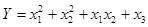(13)

500 points were generated from the equation. x1, x2, x3, x4 and x5 are assumed to be the possible influencing factors. All five variables are uniformly distributed random numbers with the statistics listed in Table 7.

Table 7. The statistics of (x1, x2, x3, x4, x5).

 Variable Range Mean Variance x1 +1~+3 1.98344 0.32414 x2 +1~+3 1.97012 0.32172 x3 +1~+3 2.02381 0.33523 x4 +1~+3 2.03522 0.33273 x5 +1~+3 2.03442 0.32308

In our study, 400 points were used for NN’s training and 100 points were used for testing. Table 8 presents the MAEs of NN modeling with different input variables. It shows that NN has the efficient learning to the system.

Table 8. The MAEs of NN modeling with different input variables.

 NN Size 3-4-1 4-4-1 5-4-1 Input Variables (x1,x2,x3) (x1,x2,x3,x4) (x1,x2,x3,x4,x5) MAE 0.022816 0.021972 0.022432

Table 9 presents ID and PID values calculated by NN models with three, four and five inputs, respectively. From the table results shown, the ratios of ID and PID of (x1, x2, x3) in three NN modeling systems are almost equal. Same as the studies in linear system, the ID and PID values of x4 and x5 are much smaller than x1, x2 and x3. In other words, the variables (x1, x2, x3) can be concluded as the real useful inputs to Y and (x4, x5) could be inferred to be the noise terms.

Table 9. ID and PID values calculated by three NN models.

 NN Size 3-4-1 4-4-1 5-4-1 x1 ID 32.54322 37.59859 37.84341 (PID) (46.03%) (45.47%) (45.62%) x2 ID 32.28733 38.13446 38.18699 (PID) (45.57%) (46.12%) (46.03%) x3 ID 5.87626 6.88912 6.78823 (PID) (8.31%) (8.33%) (8.18%) x4 ID 0.06987 0.08241 (PID) (0.08%) (0.10%) x5 ID 0.05125 (PID) (0.06%)

In fact, unlike linear modeling, no clear information about the important degree of individual input variable to output could be obtained in nonlinear modeling. However, from the PID results shown in Table 9, we found that the important degrees of x1 and x2 are almost the same. This phenomenon could be observed from Eq. (13) when both x1 and x2 have the same statistic distribution.

Similar as previous study, the nonlinear system identification by NN model is studied continuously. A nonlinear equation given by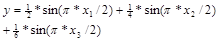(14)

1000 points were generated from the equation and then the system was assumed to be unknown. 800 points were used for NN’s training and 200 points were used for testing. Four variables, x1, x2, x3 and x4 are assumed to be the possible influencing factors to the system’s output y. Four input variables are uniformly distributed random numbers with the statistics listed in Table 10.

Table 10. The statistics of (x1, x2, x3, x4).

 Variable Range Mean Variance x1 0~+1 0.5013 0.0842 x2 0~+1 0.5088 0.0837 x3 0~+1 0.5017 0.0833 x4 0~+1 0.5115 0.0829

A size of 4-5-1 NN was used to perform the model’s identification. Table 11 presents the simulation results including MAEs of the training and test of NN and ID and PID calculations of (x1, x2, x3, x4) for NN’s modeling with four inputs.

Table 11. The simulation results of NN’s modeling for nonlinear equation.

 Training MAE Test MAE 0.00812 0.00821 Variables x1 x2 x3 x4 ID 8.1084 4.3129 2.6016 0.0953 PID 53.63% 28.53% 17.21% 0.63%

In this study, due to the ranges of (x1, x2, x3, x4) are all in [0,1], thus the angles of sine function are within the range of [0, π/2]. From the nonlinear Eq. (14), it can be observed that the important degree of (x1, x2, x3) should be (1:0.5:0.33333). From the simulation results shown, PID ratio of (x1, x2, x3, x4) is (1:0.5320:0.3209:0.0117). The PID ratio of (x1, x2, x3) is very close to the ratio (1:0.5:0.33333) of the coefficients (x1, x2, x3) in the original nonlinear equation. The ID and PID values of x4 are very small. Thus, x4 could be treated as the useless term or noise.

In above studies of NN versus nonlinear regression models, the simulation results show that NN model is also capable of obtaining the important degree of each input variable to the system output. In order to further prove our point of view, the example of real industrial system identification is continuously studied in the following section.

3.4. NN vs. Industrial System

It is known that touch panel (TP) has become an indispensable part for many electronic appliances such as computer, mobile phone, ticket vendor, etc. Usually, a thin plate with coating film will be stuck on the screen of TP as a decoration and protection film. Basically, the film’s coating process is accomplished by the evaporator under the vacuum condition. However, before the evaporation process is taken, the relevant control parameters of evaporator are needed to be set precisely in order to ensure the whole coating process could be accomplished successfully. Thus, these control parameters could be treated as the influencing factors of evaporation process. Transmittance is an important optical property of TP film. Its value is highly correlated with the influencing factors of evaporation process. The relationship between transmittance and its relevant influencing factors is very complex and nonlinear. Thus, how to use NN to find the real influencing factors of TP film’s transmittance is the aim of the study in this section.

In our research, TP film with two layers coating is studied. The coating targets are Cr and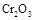. The information of data collected for the experiments includes the value of quartz crystal deposition monitor (x1), the rotation speed of holder (x2), the substrate position of panel placed (x3), Cr thickness (x4),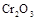thickness (x5) and TP transmittance (y). The complex relationship between y and its possible influencing factors (x1, x2, x3, x4, x5) is expected to be obtained by NN model. In order to fairly demonstrate the feasibility of NN model and the computational technique proposed, two data sets named Set-1, Set-2, are re-organized randomly from the original data base. For each data set, 100 points are used for NN’s training and 44 points are used for testing. Except MAE, the mean absolute percentage error (MAPE) is also used as the measurement of NN performance.

Table 12. The error statistics performed by five-input NN model.

 Inputs: x1, x2, x3, x4, x5 Set MAE MAPE (%) Training Test Training Test Set-1 0.6728 0.7523 1.98% 2.03% Set-2 0.5979 0.7551 1.68% 2.18% Avg. 0.6353 0.7537 1.83% 2.10%

Table 13. ID and PID values calculated by five-input NN model.

 Training Data x1 x2 x3 x4 x5 ID(Set-1) 3.290 1.104 0.441 18.162 16.911 ID(Set-2) 1.775 7.704 0.175 17.692 14.226 Avg. 2.533 4.404 0.308 17.927 15.568 PID(Set-1) 8.24% 2.77% 1.11% 45.51% 42.37% PID(Set-2) 4.27% 18.53% 0.42% 42.56% 34.22% Avg. 6.26% 10.65% 0.76% 44.03% 38.30% Test Data x1 x2 x3 x4 x5 ID(Set-1) 1.252 0.566 0.163 6.441 5.998 ID(Set-2) 0.837 3.457 0.086 8.252 6.635 Avg. 1.045 2.011 0.125 7.346 6.316 PID(Set-1) 8.68% 3.93% 1.13% 44.67% 41.59% PID(Set-2) 4.34% 17.94% 0.45% 42.83% 34.44% Avg. 6.51% 10.93% 0.79% 43.75% 38.01%

Table 12 lists the MAEs and MAPEs of transmittance estimations performed by a five-input NN model with size 5-6-1. From MAPEs shown, we conclude that NN model has the efficient training. ID and PID values for all inputs are calculated and listed in Table 13. From the values of PID shown, it is clearly found that Cr thickness (x4) and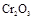thickness (x5) are two most important influencing factors to TP transmittance. The value of quartz crystal deposition monitor (x1) and the rotation speed of holder (x2) also have the certain impacts to transmittance. In these five input variables, the substrate position of panel placed (x3) could be ignored due to its small PID value.

In order to prove our conclusion, the transmittance estimations by NN model with different input combinations are redone. Table 14 lists MAEs and MAPEs of transmittance estimations performed by NN model with different four-input combinations. Table 15 lists MAEs and MAPEs of transmittance estimations performed by NN model with different three-input combinations. From the results shown in these tables, it is clearly found that the conclusion we made in accordance with the values of ID and PID is correct. In other words, the evolutionary NN technique we proposed is very promising and potential in the real applications of system identification.

Table 14. The error statistics performed by NN model with four inputs.

 Inputs: x1, x2, x4, x5 Set MAE MAPE (%) Training Test Training Test Set-1 0.7039 0.6345 2.07% 1.76% Set-2 0.6379 0.7629 1.80% 2.19% Avg. 0.6709 0.6987 1.93% 1.98% Inputs: xx1, x3, x4, x5 Set MAE MAPE (%) Training Test Training Test Set-1 0.9274 0.8724 2.94% 2.62% Set-2 0.9212 1.0875 2.82% 3.45% Avg. 0.9243 0.9799 2.88% 3.03% Inputs: x2, x3, x4, x5 Set MAE MAPE (%) Training Test Training Test Set-1 0.8204 0.8998 2.58% 2.59% Set-2 0.7274 1.0458 2.18% 3.24% Avg. 0.7739 0.9728 2.38% 2.91%

Table 15. The error statistics performed by NN model with three inputs.

 Inputs: x1, x4, x5 Set MAE MAPE (%) Training Test Training Test Set-1 0.9728 0.8387 3.08% 2.51% Set-2 0.9384 0.8968 2.96% 2.77% Avg. 0.9556 0.8677 3.02% 2.64% Inputs: x2, x4, x5 Set MAE MAPE (%) Training Test Training Test Set-1 0.7051 0.6265 2.06% 1.74% Set-2 0.6358 0.7599 1.79% 2.19% Avg. 0.6705 0.6932 1.93% 1.96% Inputs: x3, x4, x5 Set MAE MAPE (%) Training Test Training Test Set-1 1.8626 1.9028 5.60% 5.33% Set-2 1.9156 1.7562 5.65% 5.17% Avg. 1.8891 1.8295 5.63% 5.25%

4. Conclusion

This research presents an evolutionary NN technique for the application of system identification. The linear and nonlinear systems and the real industrial system were studied and simulated. The study results obviously show that the novel technique proposed indeed has its feasibility and superiority in the application of system identification. The computational methods denoted by ID and PID can extract the useful and important inputs to the system output. In other words, this study proposes a new system identification technique based on supervised NN and this technique has the potential in the area of data mining with large database.

In this research, the activation function for all nodes of NN is the sigmoid function. The ID and PID values defined are also derived from the characteristic of sigmoid function. Thus, it is believed that NN model with any node’s transfer function which has the same characteristic as sigmoid function certainly has the similar capability in the application of data mining. For instance, the hyperbolic tangent function is also a choice.

It is well known that NN with BP learning algorithm usually has the local minimum problem in its learning process. An ill-learning NN model might have the generalization and accuracy problems, if NN model really plunged into the local minimum. This condition might cause the incorrect ID and PID values calculated from the ill-trained NN model. Thus, we have to emphasize that NN model must have an efficient learning for ensuring the correct ID and PID information could be obtained.

Acknowledgements

This research was supported by the Ministry of Science and Technology, Taiwan, R.O.C. under Contracts No. MOST-105-2221-E-214-041.

References

1. M. Schetzen, "The Volterra and Wiener Theories of Nonlinear Systems". Wiley, 1980.
2. S. A. Billings, "Nonlinear System Identification: NARMAX Methods in the Time, Frequency, and Spatio-Temporal Domains". Wiley, 2013.
3. O. Nelles, "Nonlinear System Identification: From Classical Approaches to Neural Networks". Springer Verlag,2001.
4. M. Letitia, "Dynamic multivariate B-spline neural network design using orthogonal least squares algorithm for non-linear system identification", 2014 18th International Conference on System Theory, Control and Computing, ICSTCC 2014, pp. 720-725, 2014.
5. K.J. Nidhil Wilfred, S. Sreeraj, B. Vijay, V. Bagyaveereswaran, "System identification using artificial neural network", IEEE International Conference on Circuit, Power and Computing Technologies, ICCPCT 2015, July 15, 2015.
6. Hector M. Romero Ugalde, J. C. Carmona, R. R. Juan, Victor M. Alvarado, J. Mantilla, "Computational cost improvement of neural network models in black box nonlinear system identification", Neurocomputing, vol. 166, pp. 96-108, 2015.
7. Leandro L.S. Linhares, José M. Araújo, Fábio M.U. Araújo, T. Yoneyama, " A nonlinear system identification approach based on Fuzzy Wavelet Neural Network", Journal of Intelligent and Fuzzy Systems, vol. 28, no. 1, pp. 225-235, 2015.
9. L. Guan, H. J. Liang, "Data warehouse and data Mining," Microcomputer Applications, vol. 15, no. 9, pp. 17-20, 1999.
10. J. S. Feng, "KDD and its applications," BaoGang Techniques. vol. 3, pp. 27-31, 1999.
11. I. H. Witten, E. Frank, Data Mining: Practical Machine Learning, Morgan Kaufmann, 2000.
12. J. Han and M. Kamber, Data Mining: Concepts and Techniques, Morgan Kaufmann, 2001.
13. D. Hand, Principles of Data Mining, Massachusetts Institute of Technology, 2001.
14. G. Wang, D. Huang, "The summary of the data mining technology," Computer Application Technology, vol. 69, pp. 9-14, 2007.
15. R. F. Gunst, R. L. Mason, Regression Analysis and Its Application: A Data-Oriented Approach, Marcel Dekker Inc. New York, 1980.
16. G. Towell, J. W. Shavlik, "The extraction of refined rules from knowledge-based neural networks," Machine Learning, vol. 13, pp. 71-101, 1993.
17. H. Lu, R. Setiono, H. Liu, "Effective data mining using neural network," IEEE Transactions on Knowledge and Data Engineering, vol. 8, no. 6, pp. 957-961, 1996.
18. Z. Liu, L. Jing, "The research of data mining based on neural networks," Computer Engineering and Application, vol. 3, pp. 172-173, 2004.
19. S. Zhang, "Research of rule extraction and classification algorithm based on neural network," Master dissertation, Harbin Engineering University, China, 2006.
20. L. P. Duan, L. J. Zhou, Y. Wang, "Data mining based on neural networks," Techniques of Automation & Applications, vol. 7, pp. 12-19,. 2007.
21. L. Li, B. Zhang, M. Yang, "Data mining algorithm based on fuzzy neural network," Computer Engineering, vol. 33, pp. 63-64, 2007.
22. X. Ni, "Research of data mining based on neural networks," World Academy of Science, Engineering and Technology, vol. 39, pp. 381-384, 2008.
23. S. Nirkhi, "Potential use of artificial neural network in data mining," in Proc. 2nd Int. Conf. Computer and Automation (ICCAE), 2010, vol. 2, pp. 339-343.
24. P. T. Hsu, "The studies of data mining by using neural network", Master Thesis, I-Shou University, Taiwan, 2012.
25. J. C. Chien, "The practical study of neural network in data mining", Master Thesis, I-Shou University, Taiwan, 2013.

 Contents 1. 2. 3. 3.1. 3.2. 3.3. 3.4. 4.
Article ToolsAbstractPDF(339K)